# Area

Here you will learn about area, including how to calculate the area of triangles, quadrilaterals, and composite shapes.

Students will first learn about area as part of measurement and data in 3rd grade and 4 th grade. They continue to build upon this topic as a part of geometry in 6th grade and 7th grade.

## What is area?

Area is a measure of the amount of space there is inside a 2D shape.

The area of a shape is measured in square units such as \mathrm{cm}^2 ,\mathrm{~mm}^2 ,\mathrm{~m}^2 .

To calculate area, you can use different formulas depending on the shape.

Below are the formulas to find the area of common shapes.

For each of the shapes, there are also pages where you can see more examples and practice questions.

ShapeFormula

\text{Area}=\text{base} \times \text{height}

*This can also be referred to as

\text{length} \times \text{width}

Triangle

Area=\cfrac{1}{2} \times base \times height \, (perpendicular)

Step-by-step guide: Area of a square

Step-by-step guide: Area of a rectangle

Step-by-step guide: Area of a triangle

Step-by-step guide: Area of a right triangle

Step-by-step guide: Area of isosceles triangle

Step-by-step guide: Area of equilateral triangle

Step-by-step guide: Area of obtuse triangle

A composite shape can be split into two or more smaller shapes and the area of these shapes can be found. The total area of the composite shape can be found by adding the smaller areas together.

You can also find the area of composite shapes by breaking them up into triangles and rectangles and using the formulas.

\hspace{1cm} Composite Shapes (composed of triangles and rectangles)

ShapeSolving Strategy/Formula

Break the figure up into rectangles, find the area of each
and then add all the areas together.

\begin{aligned} Area &= 4 \times 9+6 \times 2 \\ &=36+12 \\ &=48 \, cm^2 \end{aligned}

Composite shapes

Break the figure up into rectangles and triangles, find the
area of each and then add all the areas together.

\begin{aligned} Area &= 11 \times 7+\cfrac{1}{2} \times 7 \times 2 \\ &=77+7\\ &=84 \, m^2 \end{aligned}

Step-by-step guide: Area of rectilinear figures

Step-by-step guide: Area of composite shapes

Parallelograms are made of a rectangle and two triangles.
Find the area of each and add them together.

\text{Area of a rectangle}=base \times height

\text{Area of a triangle}=\cfrac{1}{2} \times base \times height
\text{(perpendicular)}

If you know the base and the height, you can also
use the formula for parallelograms.

\text{Area of a parallelogram}=base \times height

A rhombus can be broken up into rectangles and triangles.
Find the area of each and add them together.

\text{Area of a rectangle}=base \times height

\text{Area of a triangle}=\cfrac{1}{2} \times base \times height
\text{(perpendicular)}

If you know the two diagonals, you can also use the
formula for a rhombus.

\text{Area of a rhombus}=\cfrac{1}{2}\, \times diagonal \times diagonal

Trapezoid

Trapezoids are made of a rectangle and one or two triangles.
Find the area of each and add them together.

\text{Area of a rectangle}=base \times height

\text{Area of a triangle}=\cfrac{1}{2} \times base \times height
\text{(perpendicular)}

If you know the two parallel side lengths and the height,
you can also use the formula for trapezoids.

\text{Area of a trapezoid}=\cfrac{1}{2} \, (a+b)h

Step-by-step guide: Area of a quadrilateral

Step-by-step guide: Area of a parallelogram

Step-by-step guide: Area of a rhombus

Step-by-step guide: Area of a trapezoid

### What is area?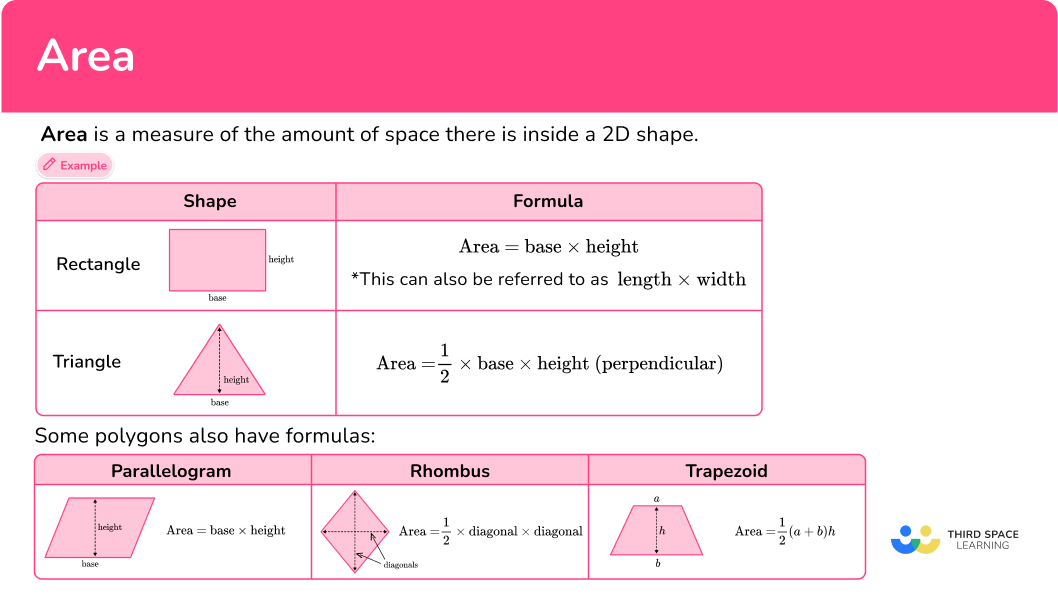## Common Core State Standards

• Grade 3 – Measurement and Data (3.MD.C.7)
Relate area to the operations of multiplication and addition.

a. Find the area of a rectangle with whole-number side lengths by tiling it, and show that the area is the same as would be found by multiplying the side lengths.

b. Multiply side lengths to find areas of rectangles with whole number side lengths in the context of solving real world and mathematical problems, and represent whole-number products as rectangular areas in mathematical reasoning.

c. Use tiling to show in a concrete case that the area of a rectangle with whole-number side lengths a and b + c is the sum of a \times b and a \times c . Use area models to represent the distributive property in mathematical reasoning.

d. Recognize area as additive. Find areas of rectilinear figures by decomposing them into non-overlapping rectangles and adding the areas of the non-overlapping parts, applying this technique to solve real world problems.

• Grade 4 – Measurement and Data (4.MD.A.3)
Apply the area and perimeter formulas for rectangles in real world and mathematical problems. For example, find the width of a rectangular room given the area of the flooring and the length, by viewing the area formula as a multiplication equation with an unknown factor.

• Grade 6 – Geometry (6.G.A.1)
Find the area of right triangles, other triangles, special quadrilaterals, and polygons by composing into rectangles or decomposing into triangles and other shapes; apply these techniques in the context of solving real-world and mathematical problems.

• Grade 7 – Geometry (7.G.B.6)
Solve real-world and mathematical problems involving area, volume and surface area of two- and three-dimensional objects composed of triangles, quadrilaterals, polygons, cubes, and right prisms.

## How to calculate area

In order to calculate the area of a shape with a formula:

1. Write down the formula for the area of the shape.
2. Identify the dimensions required for the formula.
3. Substitute the dimensions and calculate.

## Area examples

### Example 1: area of a rectangle

Find the area of this rectangle below:

1. Write down the formula for the area of the shape.

Rectangle:

\text { Area }=\text { base } \times \text { height }

2Identify the dimensions required for the formula.

\begin{aligned}& \text { Base }=7 \mathrm{~m} \\\\ & \text { Height }=4 \mathrm{~m}\end{aligned}

3Substitute the dimensions and calculate.

\begin{aligned}\text { Area } & =\text { base } \times \text { height } \\\\ & =7 \times 4 \\\\ & =28\end{aligned}

In this case, we are working with meters, so our final answer must be in square meters.

\text { Area }=28 \mathrm{~m}^{2}

### Example 2: area of a triangle

Find the area of the right triangle below:

Triangle:

\text { Area }=\cfrac{1}{2} \, \times \text { base } \times \text { height }

\begin{aligned}& \text { Base }=8 \mathrm{~cm} \\\\ & \text { Height }=6 \mathrm{~cm}\end{aligned}

\begin{aligned}\text { Area } & =\cfrac{1}{2} \, \times \text { base } \, \times \text { height } \\\\ & =\cfrac{1}{2} \, \times 8 \, \times 6 \\\\ & =24\end{aligned}

In this case, we are working with centimeters, so our final answer must be in square centimeters.

\text { Area }=24 \mathrm{~cm}^{2}

### Example 3: area of a triangle – decimals

Find the area of the isosceles triangle below:

Triangle:

\text { Area }=\cfrac{1}{2} \, \times \text { base } \, \times \text { height }

\begin{aligned}& \text { Base }=7.7 \mathrm{~m} \\\\ & \text { Height }=20.9 \mathrm{~m}\end{aligned}

\begin{aligned}\text { Area } & =\cfrac{1}{2} \, \times \text { base } \, \times \text { height } \\\\ & =\cfrac{1}{2} \, \times 7.7 \, \times 20.9 \\\\ & =80.465\end{aligned}

\text { Area }=80.465 \mathrm{~m}^2

### Example 4: area of a parallelogram

Find the area of the parallelogram below:

Parallelogram:

\text { Area }=\text { base } \times \text { height }

\text { Base }=6 \mathrm{~cm}

\text {Perpendicular height} =30 \mathrm{~mm}

All units need to be the same to calculate the area.

Let’s change 30 \, mm to 3 \, cm .

\text { Height }=3 \mathrm{~cm}

\begin{aligned}\text { Area }&= \text { base } \times \text { height } \\\\ &=6 \times 3 \\\\ &=18\end{aligned}

\text { Area }=18 \mathrm{~cm}^{2}

### Example 5: area of a trapezoid

Find the area of the trapezoid below:

This trapezoid is made up of two congruent triangles and a rectangle.

Rectangle:

\text { Area }=\text { base } \times \text { height }

Triangle:

\text { A }=\cfrac{1}{2} \times \text { base } \times \text { height }

Rectangle:

\begin{aligned}& \text { Base }=5 \mathrm{~m} \\\\ & \text { Height }=4 \mathrm{~m}\end{aligned}

Triangle:

\begin{aligned} & \text { Base }=2 \mathrm{~m} \\\\ & \text { Height }=4 \mathrm{~m} \end{aligned}

Rectangle:

\begin{aligned}\text { Area } & =\text { base } \times \text { height } \\\\ & =5 \times 4 \\\\ & =20\end{aligned}

Triangle:

\begin{aligned}\text { Area } & =\cfrac{1}{2} \, \times \text { base } \times \text { height } \\\\ & =\cfrac{1}{2} \, \times 2 \times 4 \\\\ & =4 \end{aligned}

Add the area of the rectangle and the two triangles together:

20 + 4 + 4 = 28

This can also be solved with the formula for trapezoids – since the two bases and height are given.

\begin{aligned}& \text { Area }=\cfrac{1}{2} \, (a+b) h \\\\ & \text { Base } A=5 \mathrm{~cm} \\\\ & \text { Base } B=9 \mathrm{~cm} \\\\ & \text { Height }=4 \mathrm{~cm} \\\\ \end{aligned}

\begin{aligned}\text { Area } & =\cfrac{1}{2} \, (5+9) 4 \\\\ & =\cfrac{1}{2} \,(14) \, 4 \\\\ & =28\end{aligned}

\text {Area }=28 \mathrm{~m}^{2}

### Example 6: area of a composite shape – word problem

Find the area of the composite shape below:

The floor shown below needs to be tiled. Each pack of tile covers a distance of 12 square ft. How many packs of tile will be needed to cover the entire floor?

Split the composite shape into three rectangles and label them A, B and C .

Rectangle:

\text { Area }=\text { base } \times \text { height }

Notice that rectangle A and C each have a tick mark at the bottom. This means that these sides are congruent, or equal. Therefore, we know the width of rectangle A is 5.5ft .

Rectangle A :

Length=13 \mathrm{ft}

Width =5.5 \mathrm{ft}

Rectangle C is congruent to Rectangle A , so the dimensions are the same.

Rectangle B :

You need to calculate the unknown width. To do this, use the measurements you are given in the question that are parallel to the unknown side:

The entire width of the shape is 13ft .

Subtract 5.5ft and 5.5ft (the width of rectangles A and C ) to find the missing width of rectangle B: 13 - 5.5 - 5.5 = 2ft .

\begin{aligned}& \text { Length }=9 \mathrm{ft} \\\\ & \text { Width }=2 \mathrm{ft}\end{aligned}

Area of Rectangle A and C :

\begin{aligned}\text { Area } & =\text { length } \times \text { width } \\\\ & =13 \times 5.5 \\\\ & =71.5\end{aligned}

Area of Rectangle B:

\begin{aligned}\text { Area } & =\text { length } \times \text { width } \\\\ & =9 \times 2 \\\\ & =18\end{aligned}

\begin{aligned}\text { Composite Area }&= \text { Area of } A+\text { Area of } B+\text { Area of } C \\\\ & =71.5+18+71.5 \\\\ & =161\end{aligned}

\text { Composite Area }=161 \mathrm{ft}^2

Since each pack of tiles covers 12 square ft, divide the composite area by 12 to see how many packs are needed.

161 \div 12=13.416

Since a part of a pack cannot be bought, it will take 14 packs to cover the floor.

### Teaching tips for area

• Before learning the area formulas, allow students to explore the area by tiling with square tiles or by using shapes that are shown on square grids. This will help students build an understanding of area before they begin calculating it.

• Rather than having students practice finding the area with just worksheets, give them a variety of activities and projects that have a real-world context.

• Be sure to expose students to all types of triangles (acute, right and obtuse) and quadrilaterals in all different orientations when working with area. It is important that students can learn to identify the base and the height of a shape no matter its position. Always look for worksheets or study materials that provide this type of variety.

• For common shapes and composite shapes, allow students opportunities to create their own (either on paper, using manipulatives, or using an interactive website) and have a partner find the area.

### Easy mistakes to make

• Using the incorrect unit of area or not including units
Area calculations are always measured in square units.
For example,
Square millimeters (\mathrm{mm}^2) , square centimeters (\mathrm{cm}^2) , square meters (\mathrm{m}^2) , square kilometers (\mathrm{km}^2) , square inches (\mathrm{inch}^2) , square feet (\mathrm{ft}^2) , square yards (\mathrm{yd}^2) , square miles (\mathrm{mi}^2) , etc.

• Using an incorrect area formula
There are several different area formulas for the different shapes – make sure you use the correct one.

• Confusing perimeter, area and volume
Two dimensional shapes have a perimeter and an area. Three dimensional figures have a surface area and a volume. It is easy to confuse these terms and their meaning, if you don’t have a deep understanding of what they are.
For example,

• Calculating with different units
All measurements must be in the same units before calculating the area.
For example,

To find the area of this rectangle, the units need to be all ft or all inches .
Since 24 \text { inches}=2 \mathrm{ft} , the dimensions 5ft and 2ft can be multiplied to calculate the area of this rectangle in feet (10 \mathrm{ft}^2) .

### Practice area questions

1. Find the area of the rectangle.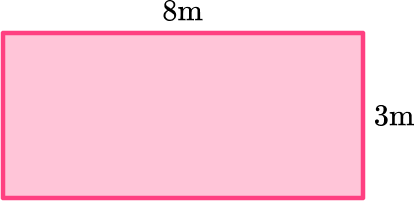11 \mathrm{~m}^222 \mathrm{~m}^224 \mathrm{~m}^212 \mathrm{~m}^2Use the formula:

\text{Area }= \text{ base } \times \text{ height}

8 \times 3=24\mathrm{~cm}^{2}

2. Find the area of the triangle.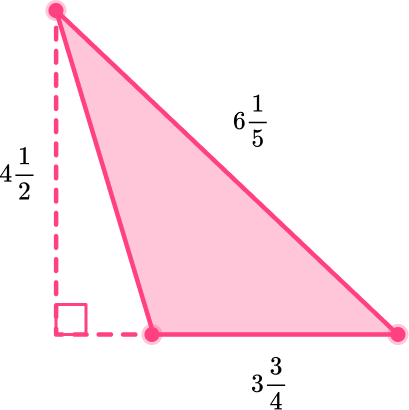8 \, \cfrac{7}{16} \text { units }^216 \, \cfrac{7}{8} \text { units }^223 \, \cfrac{5}{20} \text { units }^2209 \, \cfrac{5}{20} \text { units }^2The height of the triangle is always perpendicular to the base.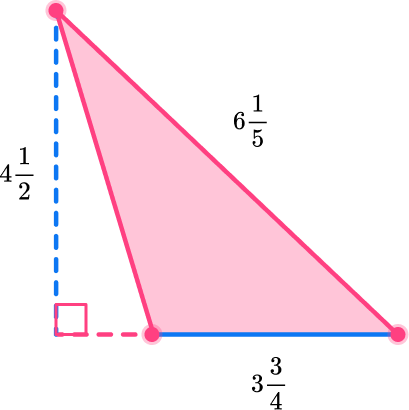\text { Base }=3 \cfrac{3}{4}

\text { Height } =4 \cfrac{1}{2}

\begin{aligned}A & =\cfrac{1}{2} \, \times \text { base } \times \text { height } \\\\ & =\cfrac{1}{2} \times 3 \cfrac{3}{4} \, \times 4 \cfrac{1}{2} \\\\ & =\cfrac{1}{2} \times \cfrac{15}{4} \, \times \cfrac{9}{2} \\\\ & =\cfrac{135}{16} \\\\ & =8 \cfrac{7}{16} \text { units }^2\end{aligned}

3. Find the area of the triangle.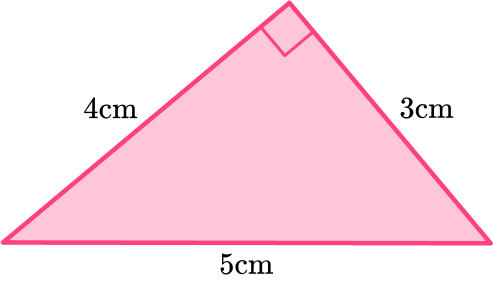12 \mathrm{~cm}^210 \mathrm{~cm}^27.5 \mathrm{~m}^26 \mathrm{~cm}^2The height of the triangle is always perpendicular to the base.

Rotate the triangle to better see the height or look for the square right angle symbol.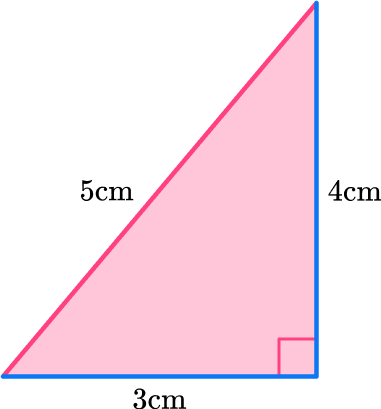Height =4 \mathrm{~cm}

Base =3 \mathrm{~cm}

\begin{aligned}A&=\cfrac{1}{2} \, \times \text{base} \times {height} \\\\ &=\cfrac{1}{2} \,\times \, 4\times 3\\\\ &=6 \mathrm{~cm}^{2}\\\end{aligned}

4. Find the area of the parallelogram in \mathrm{m}^2.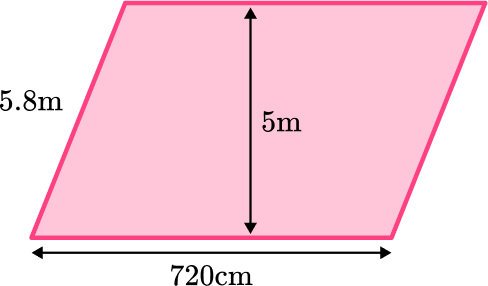36 \mathrm{~m}^241.76 \mathrm{~m}^229 \mathrm{~m}^23,600 \mathrm{~m}^2720 \mathrm{~cm}=7.2 \mathrm{~m}

\begin{aligned} & \text { Base }=7.2 \mathrm{~m} \\\\ & \text { Height }=5 \mathrm{~m}\end{aligned}

*Be careful not to confuse the perpendicular height with the diagonal side.

\begin{aligned}\text { Area } & =\text { base } \times \text { height } \\\\ & =7.2 \times 5 \\\\ & =36 \mathrm{~m}^2\end{aligned}

5. Find the area of the trapezoid below in \mathrm{cm}^2.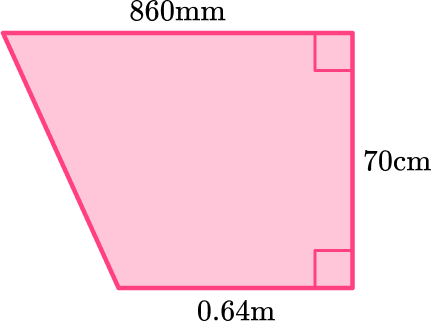6,020 \mathrm{~cm}^25,250 \mathrm{~cm}^24,480 \mathrm{~cm}^25,762 \mathrm{~cm}^2Convert all units to centimeters. Convert 0.64 \, m into 64 \, cm and 860 \, mm into 86 \, cm .

The trapezoid is made up of a rectangle and a triangle.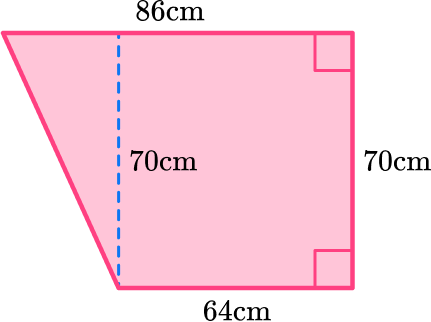For the rectangle, use the formula:

\begin{aligned}\text { Area } & =\text { base } \times \text { height } \\\\ & =64 \times 70 \\\\ & =4,480\end{aligned}

To find the area of the triangle, you need to find the base length. To do this, use the measurements you are given in the question that are parallel to the unknown side.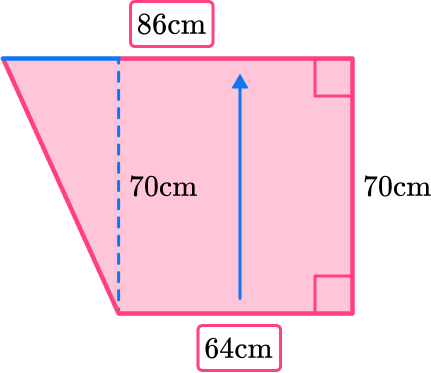The opposite side of the square is also 64 \, cm . If the entire side is  86 \, cm , subtract 64 from  86 to find the missing base length.

86 \, – \, 64 = 22

For the triangle, use the formula:

\begin{aligned}\text { Area } & =\cfrac{1}{2} \, \times \text { base } \times \text { height } \\\\ & =\cfrac{1}{2} \, \times 22 \times 70 \\\\ & =770\end{aligned}

Add the area of the rectangle and triangle together, for the total area of the trapezoid:

4,480+770=5,250 \mathrm{~cm}^2

This can also be solved with the formula.

\begin{aligned}\text{Area }&=\cfrac{1}{2}(a+b)h\\\\ &=\cfrac{1}{2} \, \times (64+86) \times 70\\\\ &=\cfrac{1}{2} \, \times 150 \times 70\\\\ &=5250 \mathrm{~cm}^{2}\end{aligned}

6. Below is the front side of a piece of wood that needs to be covered in paint. Joey has   45.34 {~cm}^2 of paint. How much more does he need to cover the front side?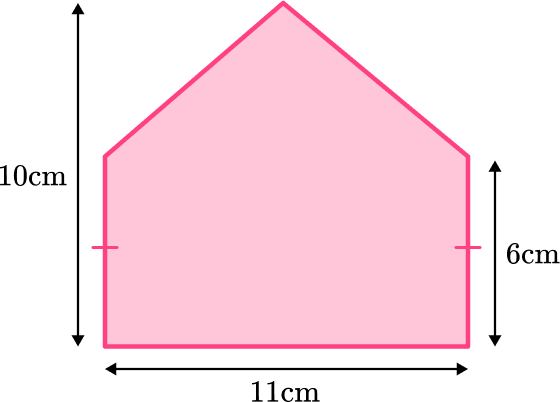88 \mathrm{~cm}^2110 \mathrm{~cm}^253.5 \mathrm{~cm}^242.66 \mathrm{~cm}^2The pentagon is made up of a rectangle and a triangle.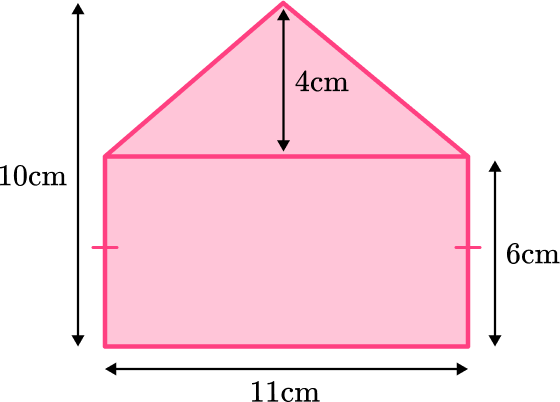\begin{aligned}\text{Area of rectangle }&=\text{ base } \times \text{ height}\\\\ &=11 \times 6\\\\ &=66 \mathrm{~cm}^{2}\end{aligned}

\begin{aligned}\text{Area of triangle }&=\cfrac{1}{2} \, \times \text{ base } \times \text{ height }\\\\ &=\cfrac{1}{2} \, \times 11 \times 4\\\\ &=22 \mathrm{~cm}^{2}\end{aligned}

Total area:  66+22=88 \mathrm{~cm}^{2}

To find how much more paint Joey needs, subtract what he has from the total amount needed.

88 \, – \, 45.34 = 42.66

Joey needs 42.66 \mathrm{~cm}^{2} more of paint to cover the front side

## Area FAQs

What is the definition of area?

Area is the measurement of the space inside of a 2D closed figure.

Can you find the area of irregular shapes?

Yes. Some irregular shapes have formulas, like rectangles and triangles. However, many do not. If an irregular shape does not have a formula, it can be broken up into simple shapes (like rectangles or triangles), finding the area of each part and then adding them all together to find the total area of the irregular shape.

Does the area of the circle have a formula?

Yes, the area of a circle is found with the formula \Pi r^2 . This is taught in 7th grade, per the Common Core State Standards.

What are synonyms for ‘2D shapes’?

They are also referred to as ‘closed figures’ or ‘plane figures.’

## Still stuck?

At Third Space Learning, we specialize in helping teachers and school leaders to provide personalized math support for more of their students through high-quality, online one-on-one math tutoring delivered by subject experts.

Each week, our tutors support thousands of students who are at risk of not meeting their grade-level expectations, and help accelerate their progress and boost their confidence.